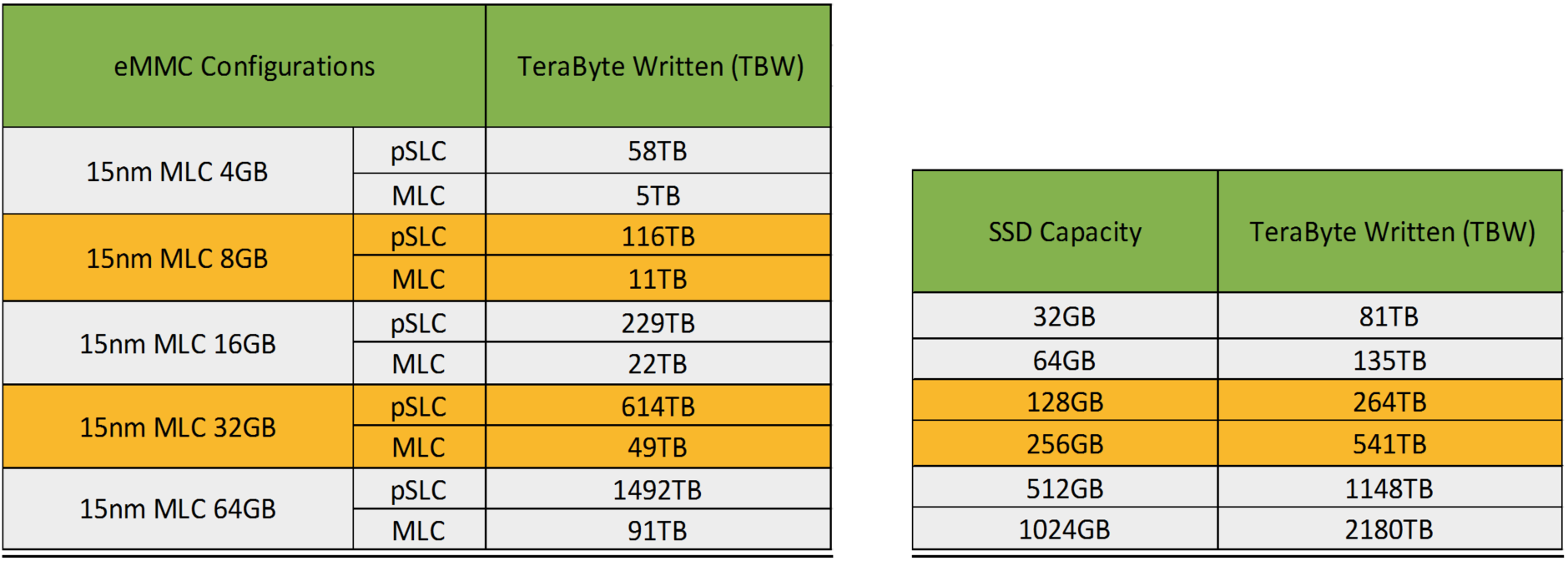* Klicken Sie hier um auf die nächste Seite zu kommen.

## Approximations of Insignis SSD & eMMC Expected Lifespans

JEDEC defined the endurance rating TBW (TeraByte Written) for indicating the number of terabytes an SSD can be written. This is an approximation of an SSD’s expected lifespan. To calculate the TBW of an SSD, the following equation is applied:

• TBW = [(NAND Endurance) x (SSD Capacity)] / WAF

NAND Endurance:

• NAND endurance refers to the P/E (Program/Erase) cycle of a NAND flash.

SSD Capacity:

• The SSD capacity is the specific capacity in total of a SSD.

Write Amplification Factor (WAF) is a numerical value representing the ratio between the amount of data that a SSD controller needs to write and the amount of data that the host’s flash controller writes. A better WAF, which is near 1, guarantees better endurance and lower frequency of data written to flash memory. The equation to calculate the WAF is:

• WAF = (Lifetime write to flash) / (Lifetime write to host)